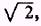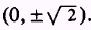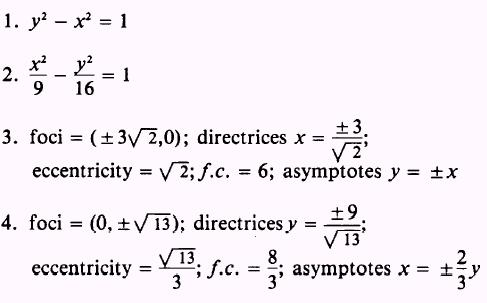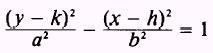Custom SearchPRACTICE PROBLEMS: 1. Find the equation of the hyperbola with an eccentricity of, directrices, and foci at2. Find the equation of the hyperbola with an eccentricity of 5/3, foci at ( 5,0), and directrices x = 9/5. Find the foci, directrices, eccentricity, equations of the asymptotes, and length of the focal chord of the hyperbolas given in problems 3 and 4.ANSWERS:The hyperbola can be represented by an equation in the general form Axe+Cy2+Dx+Ey+F=0 where the capital letters refer to independent constants and A and C have different signs. These equations can be reduced to standard form in the same manner in which similar equations for the ellipse were reduced to standard form. The standard forms with the center at (h,k) are given by the equationsandIntegrated Publishing, Inc. - A (SDVOSB) Service Disabled Veteran Owned Small Business